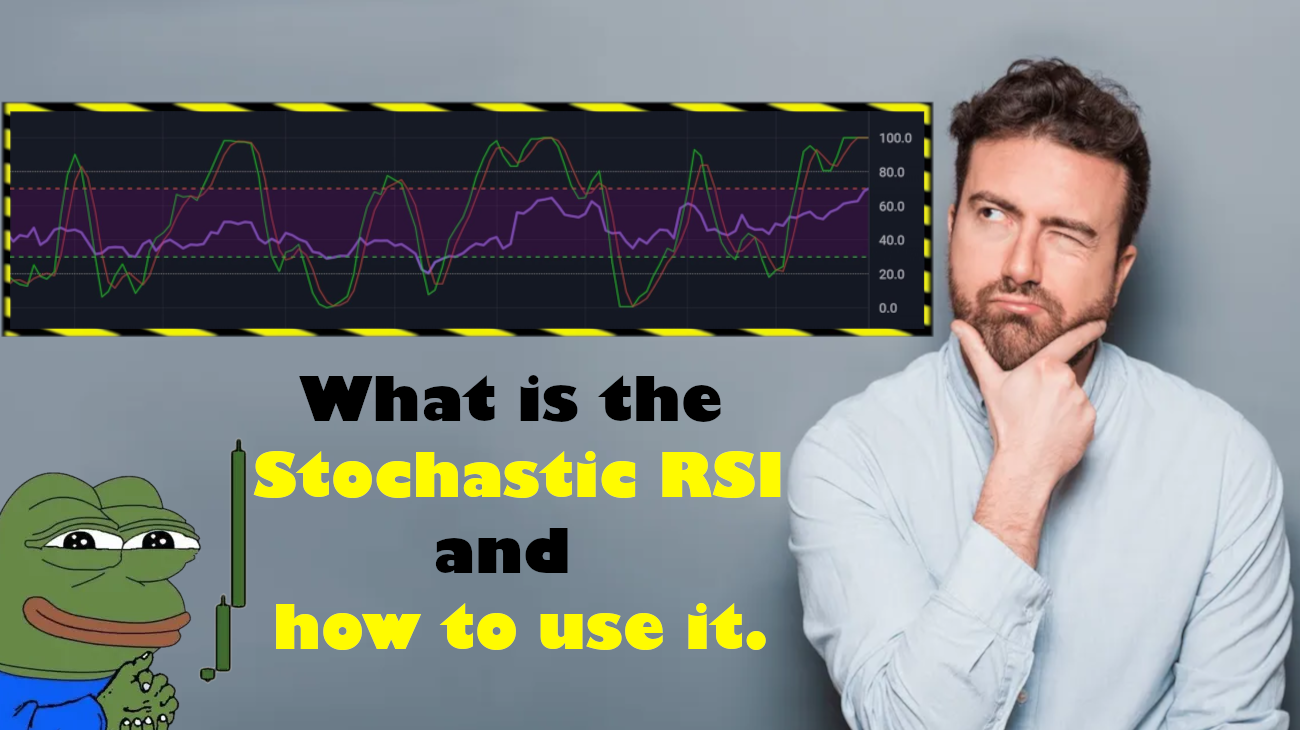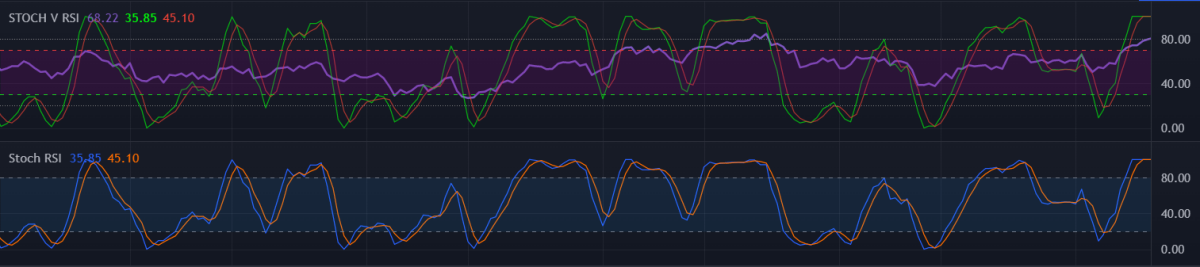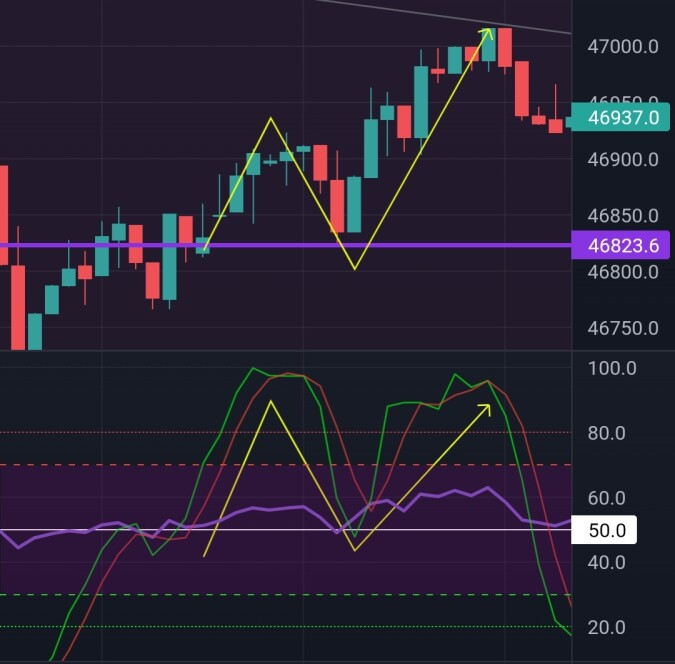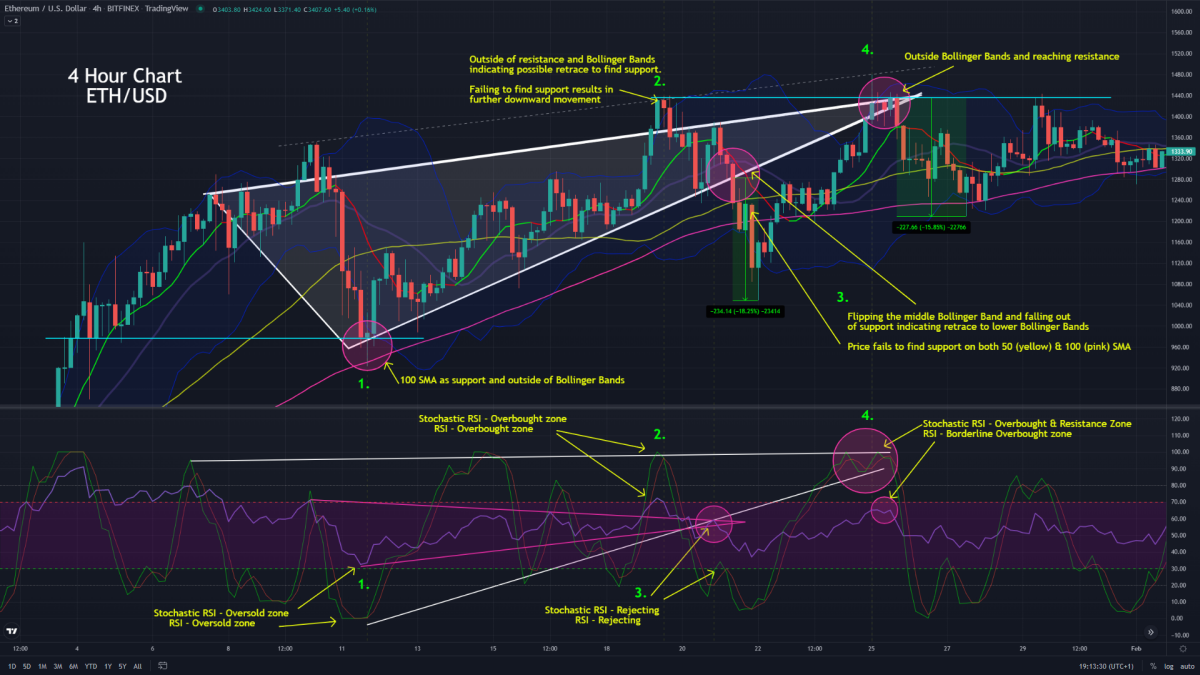# Stochastic RSI - How to use it and how it works - (with charts)

By inconspicuous | Helpful Crypto | 29 Mar 2022

## - (Stochastic Relative Strength Index) -The picture above shows both the RSI stacked with the Stochastic RSI in one indicator (top) and the Standard Stochastic RSI indicator (Bottom)

The Stochastic RSI was created to increase the sensitivity of the already RSI indicator.

The Stochastic RSI is generally set to a 14 bar/period regardless of time frame, although this can be changed to traders specifics and strategy

4h = Past 14 bars = 2d:8h
1h = Past 14 bars = 14 Hours
15m = Past 14 bars = 3h:30m
5m = Past 14 bars = 1h:10m

Both said indicators are oscillators used for identifying overbought and oversold areas by measuring price of an asset over a given time period

Oversold  (20)
An assent will be considered oversold when the Stochastic RSI shows a reading below 20
This would normally happen after downwards⬇️ momentum

Overbought  (80)
An assent will be considered overbought when the Stochastic RSI shows a reading above 80
This would normally happen after upwards⬆️ momentum

Middle  (50)
If the reading retraces to the middle and acts as support then this can indicate strength where current momentum can still be in play

• Example - Price is in an uptrend and the Stochastic RSI has been ranging in the overbought area (80+) and declines to 50 but steadily holds the 50. This can indicate support in the momentum and a possible continuation in the trend and vice versa

The yellow line on both price chart and Stochastic RSI chart (pictured below) represents trend movement with a retrace to support (purple⁴⁶⁸²³ support on chart and white 50 support on the Stochastic RSI)

Using multiple indicating levels to establish a better decision on the markets movement is what the Stochastic RSI is perfect for

*This specific indicator has stacked both RSI (Faded Purple) and Stochastic RSI (Green and red lines)

####- What is the Stochastic RSI used for and is it better than the normal RSI? -

Uses
• Identifying market trends
• Finding oversold and overbought areas
• Finding entry and exit points
• Finding price reversals
• Observing quicker trends in the market than RSI

Is it better than the RSI
Yes and no. It really depends on your strategy so its best to use an indicator that show both the RSI and Stochastic RSI together. This gives you a much better overall idea to better your decisions.

Like the EMA is to the SMA, The Stochastic RSI is a quicker and more sensitive indicator than the RSI.
It too is prone to fakeouts and isn't as reliable as its other, but can still be used well to find a change in momentum beforehand. Its also best used with other indicators to help better decisions.

##### Here is an example

You can see here how the indicators and trendlines used with both the RSI and Stochastic RSI can help give you a much better outlook on the market and give you more reasons as to why price is behaving in the way that it is. Its very important to understand you can't just use the RSI indicators alone. Decisions are exponentially improved with additional information#### - How is it calculated -

Example
Within your set period (mostly 14 bars) say that the start of the price (1st bar out of 14) starts at \$50. Price continues up but retraces at \$100 and on the 14th candle price closes at \$90.

So...
Start/Low = 50
High    =  100
Close =  90

1. Take the range from the low to the close
90-50=40    Range = 40

2. Take the range from the high to the low
100-50=50    Range = 50

3. Then dived the total range from each equation
40÷50=0.8

4. Times the total by 100 to find Stochastic RSI reading
0.8×100=80

Equation
Low - Close = x
High - Low = y
x ÷ y = z
z × 100 = Ans

#WAGMI